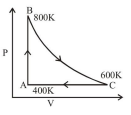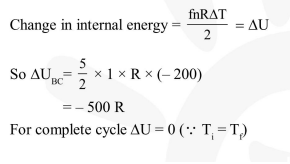Deepak Scored 45->99%ile with Bounce Back Crack Course. You can do it too!

# One mole of diatomic ideal gas undergoes a cyclic process

Question:

One mole of diatomic ideal gas undergoes a cyclic process $\mathrm{ABC}$ as shown in figure. The process $\mathrm{BC}$ is adiabatic. The temperatures at $\mathrm{A}, \mathrm{B}$ and $\mathrm{C}$ are $400 \mathrm{~K}, 800 \mathrm{~K}$ and $600 \mathrm{~K}$ respectively. Choose the correct statement :1. The change in internal energy in the process $\mathrm{AB}$ is $-350 \mathrm{R}$.

2. The change in internal energy in the process $B C$ is $-500 R$

3. The change in internal energy in whole cyclic process is $250 \mathrm{R}$.

4. The change in internal energy in the process CA is $700 \mathrm{R}$.

Correct Option: , 2

Solution: